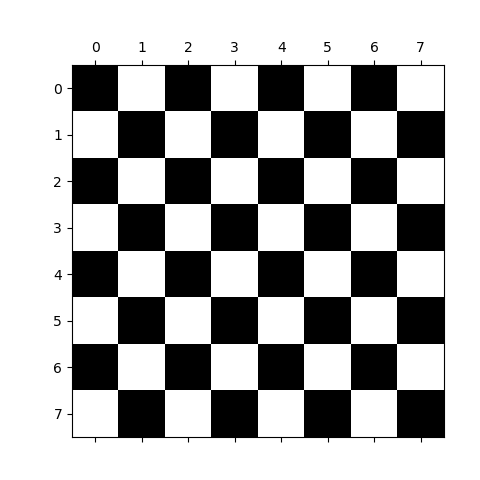# 3.3.9.1. Creating an image¶

How to create an image with basic NumPy commands : `np.zeros`, slicing…

This examples show how to create a simple checkerboard.```import numpy as np
import matplotlib.pyplot as plt

check = np.zeros((8, 8))
check[::2, 1::2] = 1
check[1::2, ::2] = 1
plt.matshow(check, cmap='gray')
plt.show()
```

Total running time of the script: ( 0 minutes 0.011 seconds)

Gallery generated by Sphinx-Gallery